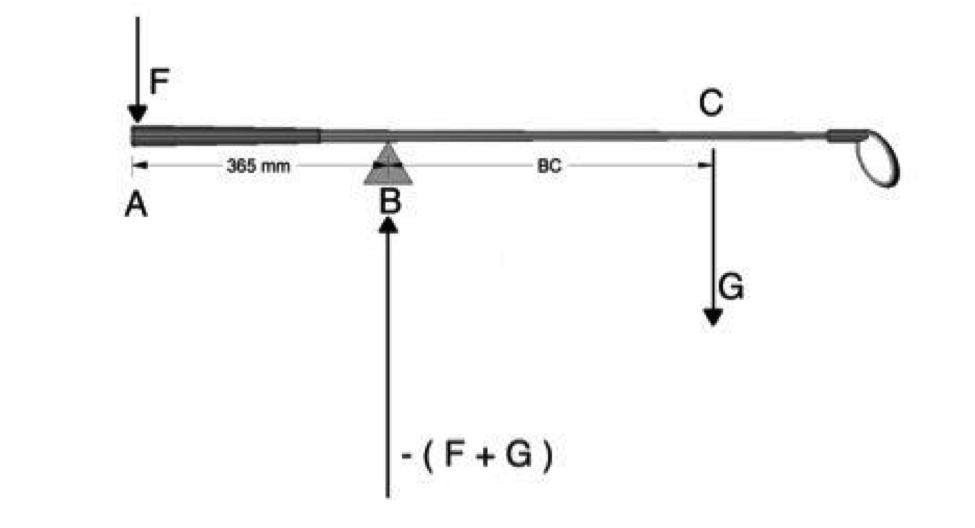# DDDD

## Rational Blog: Thoughts on Golf and the World

How to Calculate Swingweight with a Measuring Tape

A drawing showing the forces acting on a golf club positioned in a Swingweight Scale is provided bellow. The three forces acting on the golf club are as follows:
The force F, acting downwards at the grip end of the club. This is the force measured by the Swingweight instrument.
The force G is the gravitational force acting on the golf club. It is simply the weight of the golf club acting at its center of gravity. The location of the center of gravity can be determined by balancing the club on a knife-edge. The center of gravity is located at distance BC from the fulcrum.
The third force is acting upward at the fulcrum. The fulcrum is located 356 mm (14 inches) from the grip end of the club. This force equals the sum of force G and force F, acting in the opposite direction.
From Newton´s Laws of Motion, it is established that the sum of all moments acting upon a body at rest is zero. By adding the moments acting on the golf club about the fulcrum in the Swingweight instrument, denoting clockwise as the positive direction:
Sum of all moments = ∑M = (G x AB) - (F x 356 mm) = 0
Thereby;

F = G x AB / 356 mm

The Swingweight of any golf club can thereby be calculated when the mass and center of gravity is known. Swingweight is purely a function of mass and center of gravity of the golf club. For example, a golf club is found to have a mass of 349.8 grams and a center of gravity located 824 mm from the grip end of the club. These measurements were carried out on an actual golf club using a scale, a knife-edge, and a measuring tape. From Newton´s second law of motion, the vector force G = 0.3498 Kg x 9.81 m/s^2 = 3.431 N. The distance AB = 824 mm – 356 mm = 468 mm = 0.468 m. Hence;
F = 3.431 N x 0.468 m / 0.356 m = 4.510 N
From a table that I will forward next, you can look up this number and see the Swingweight.